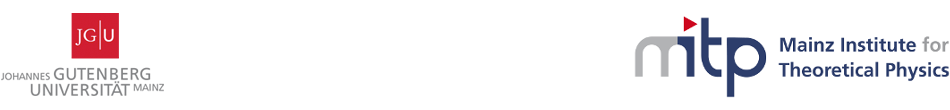#Geometry, Gravity and Supersymmetry

24-28 April 2017
Mainz Institute for Theoretical Physics, Johannes Gutenberg University
Europe/Berlin timezone

## A Riemann-Hilbert approach to attractor geometries and extremal black holes.

25 Apr 2017, 11:05
45m
02.430 (Mainz Institute for Theoretical Physics, Johannes Gutenberg University)

### 02.430

#### Mainz Institute for Theoretical Physics, Johannes Gutenberg University

Staudingerweg 9 / 2nd floor, 55128 Mainz

### Speaker

Dr Thomas Mohaupt (Department of Mathematical Sciences, University of Liverpool)

### Description

We review in which sense the stationary-axisymmetric sector of four- and five-dimensional gravity is integrable, and how the non-linear equations of motion can be viewed as the integrability conditions of a linear system. Solving this linear system can in turn be formulated as a matrix Riemann-Hilbert factorisation problem. While this has been known (at least) since the seminal work of Breitenlohner and Maison in the late 1980's, explicit solutions of Riemann-Hilbert problems are hard to obtain, and have so far been restricted to cases where the space-time is asymptotically flat, and where the monodromy matrix has first order poles in the spectral parameter. We present an efficient method for obtaining explicit matrix factorisations which avoids these assumptions, and show that the factorisation of monodromy matrices with second order poles leads to attractor geometries (near horizon geometries of extremal black holes) and extremal black hole solutions. Examples include both over-rotating and under-rotating extremal black holes and their near-horizon geometries. We also study the action of the Geroch group on monodromy matrices and on solutions of the linear system. Group elements which realise Harrison-type transformations relate attractor geometries to the corresponding extremal black hole solutions. A family of consistent non-trivial deformations of the monodromy matrix is shown to correspond to a family of Geroch group elements depending explicitly on the spectral parameter.

### Primary author

Dr Thomas Mohaupt (Department of Mathematical Sciences, University of Liverpool)

 Slides